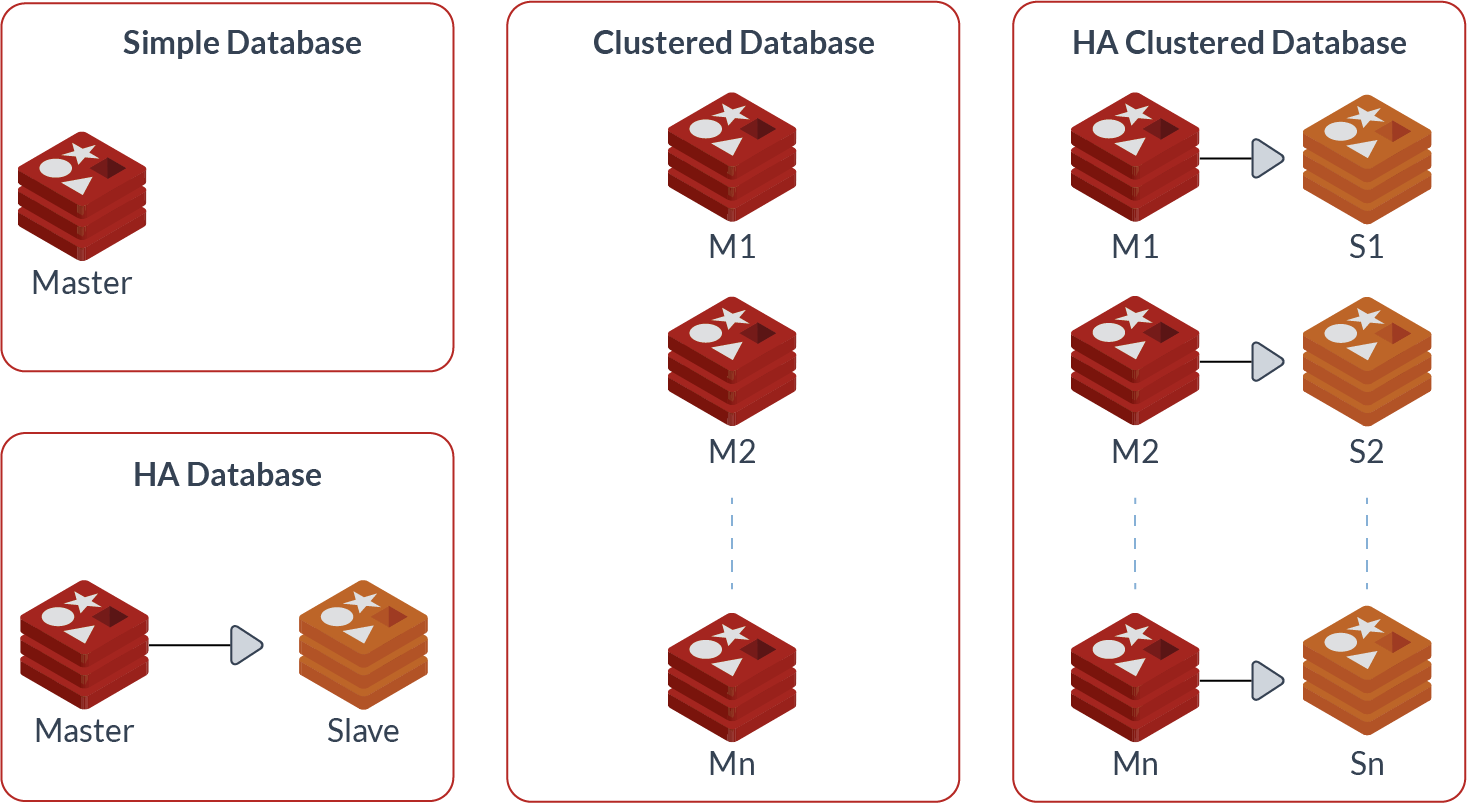## 取模哈希

``server := serverList[hash(key) % n]``

## 一致性哈希的具体实现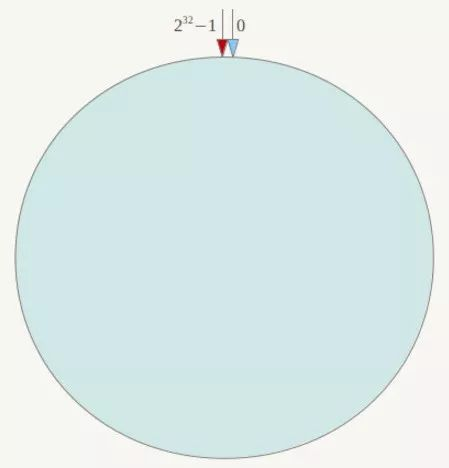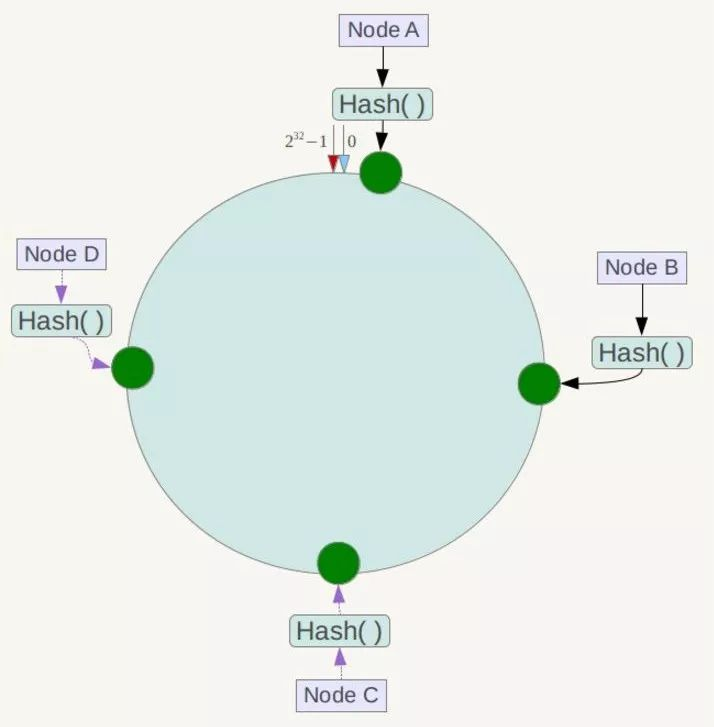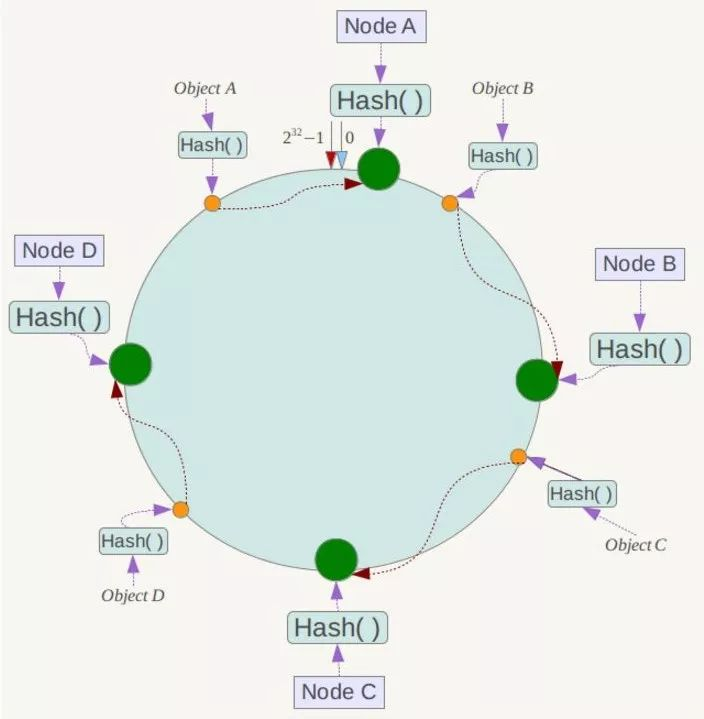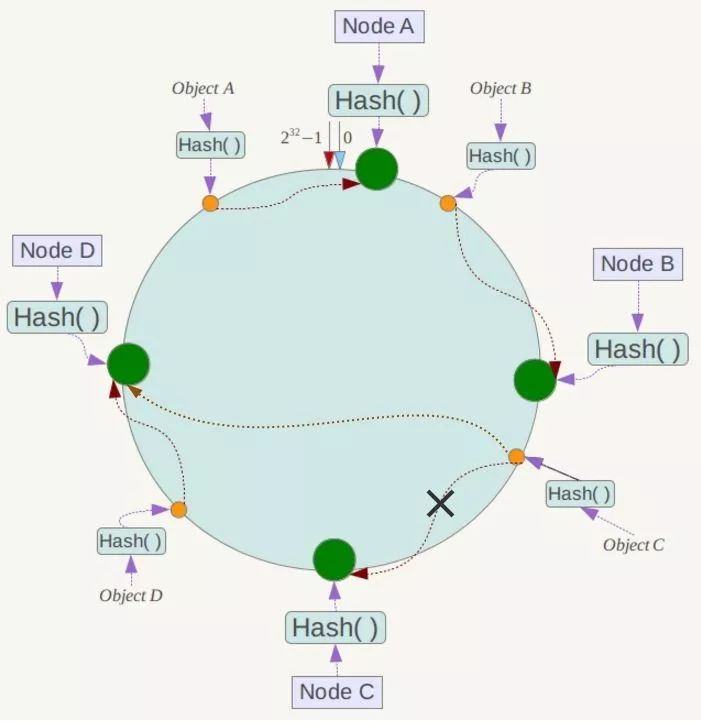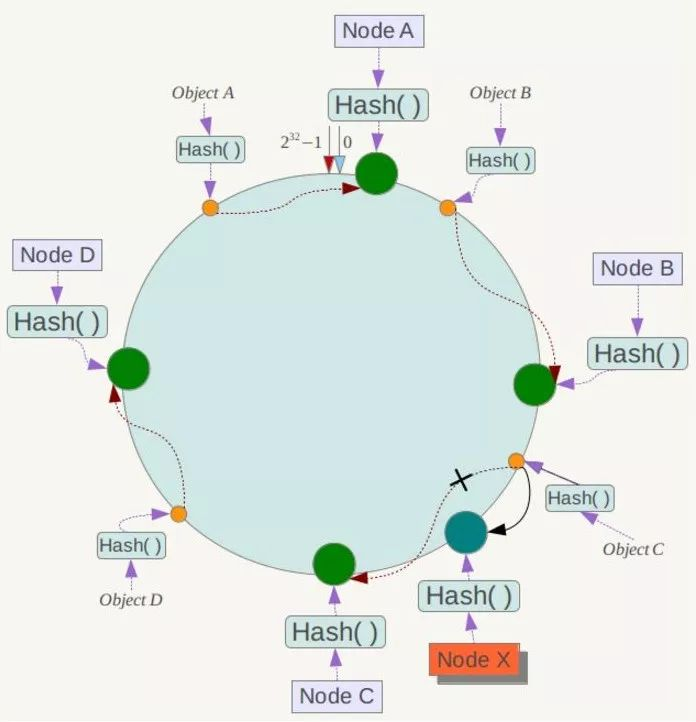## 一致性哈希虚拟节点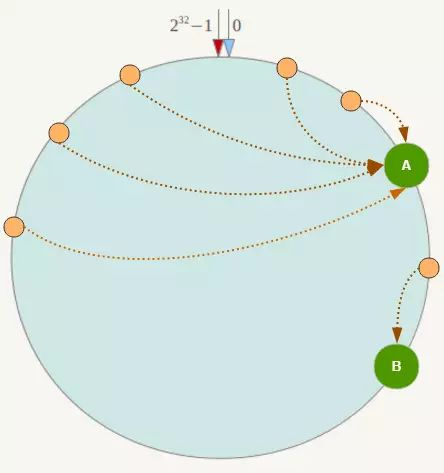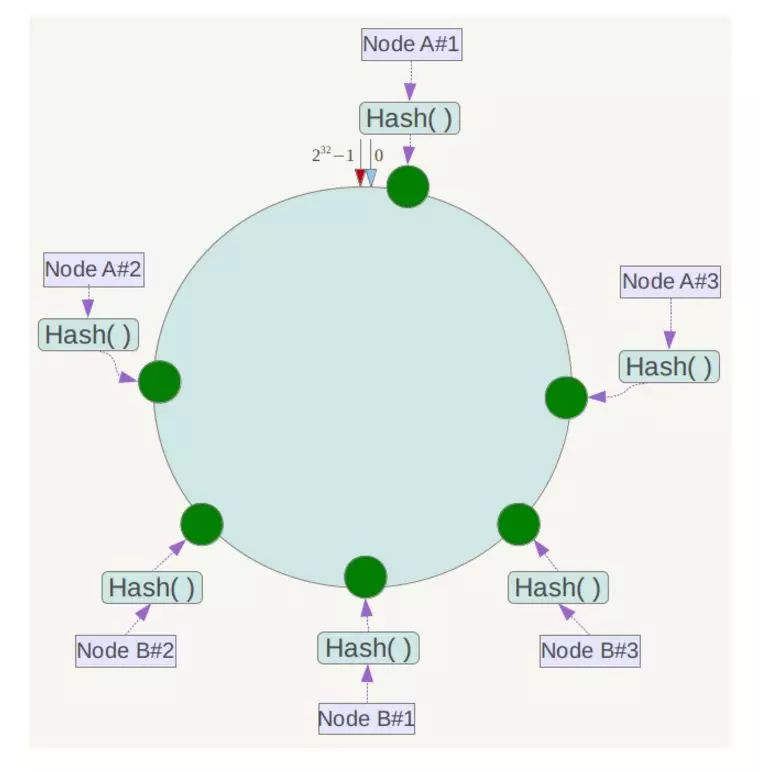## 一致性哈希代码实现

``````func (m *Map) Add(nodes ...string) {
for _, n := range nodes {
for i := 0; i < m.replicas; i++ {
hash := int(m.hash([]byte(strconv.Itoa(i) + " " + n)))
m.nodes = append(m.nodes, hash)
m.hashMap[hash] = n
}
}
sort.Ints(m.nodes)
}``````
``````func (m *Map) Get(key string) string {
hash := int(m.hash([]byte(key)))
idx := sort.Search(len(m.keys),
func(i int) bool { return m.keys[i] >= hash }
)
if idx == len(m.keys) {
idx = 0
}
return m.hashMap[m.keys[idx]]
}``````